Concepts

Computer Science - Class 11
Chapter 2 Class 11 - Encoding Schemes and Number System

## Number System Conversions

### 1. Decimal to Binary conversion

Repeatedly divide the decimal number by 2 until the quotient becomes 1 and record all the remainders. The remainders should be written bottom to upwards to get the binary equivalent of the decimal number.

Example:

(125) 10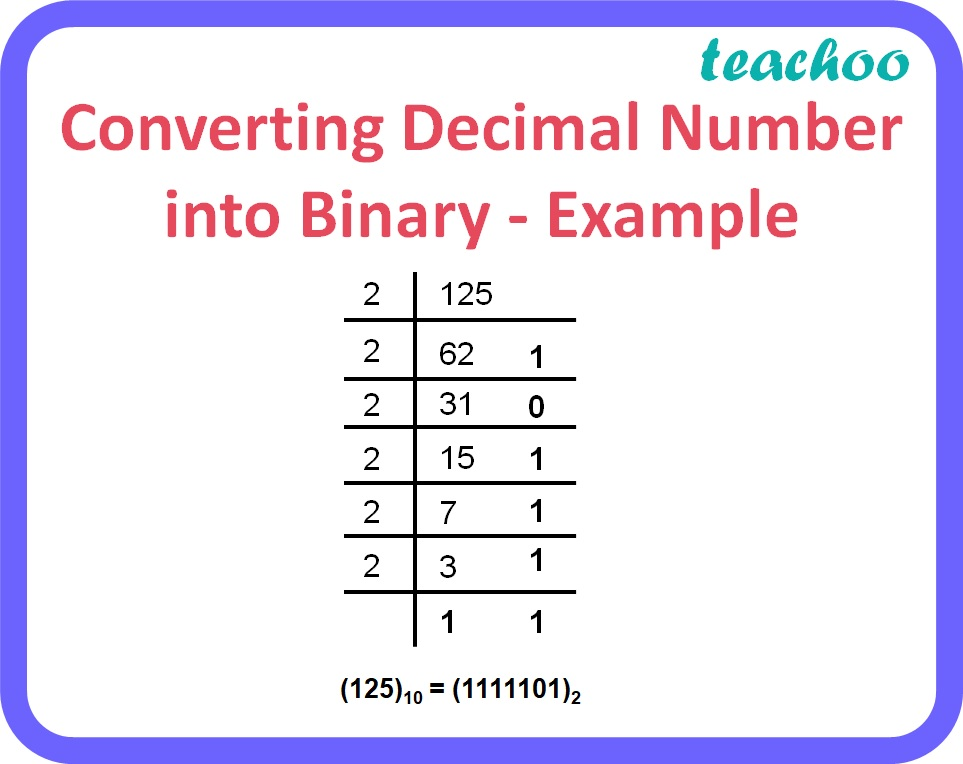(125) 10 = (1111101) 2

### 2. Decimal to octal conversion

Repeatedly divide the decimal number by 8 until the quotient becomes less than 8 and record all the remainders. The remainders should be written bottom to upwards to get the octal equivalent of the decimal number.

Example:

(973) 10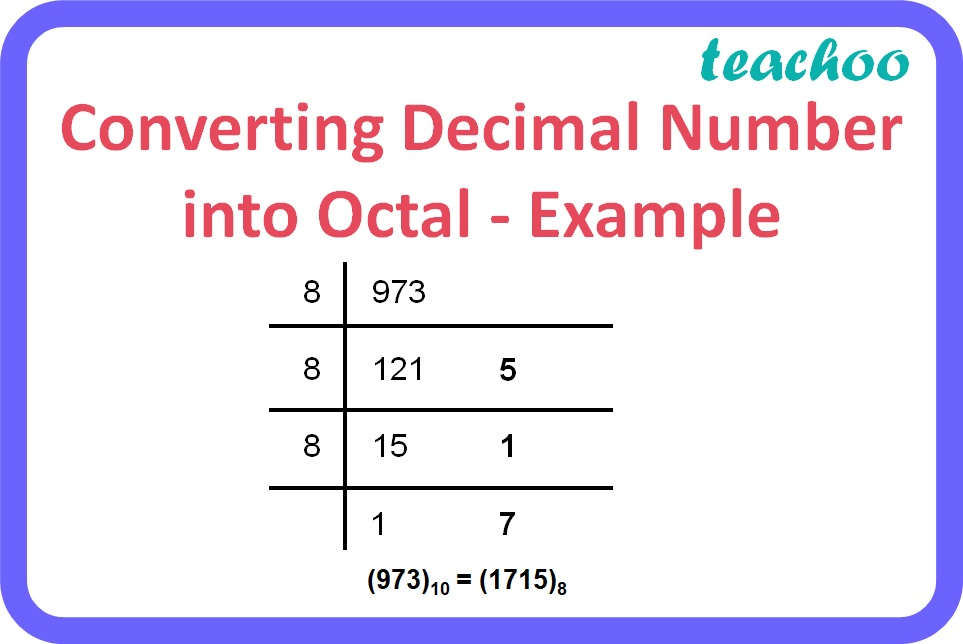(973) 10 = (1715) 8

### 3. Decimal to hexadecimal conversion

Repeatedly divide the decimal number by 16 until the quotient becomes less than 16 and record all the remainders. The remainders should be written bottom to upwards to get the hexadecimal equivalent of the decimal number.

Here,

• 10 = A
• 11 = B
• 12 = C
• 13 = D
• 14 = E
• 15 = F

Example:

(1973) 10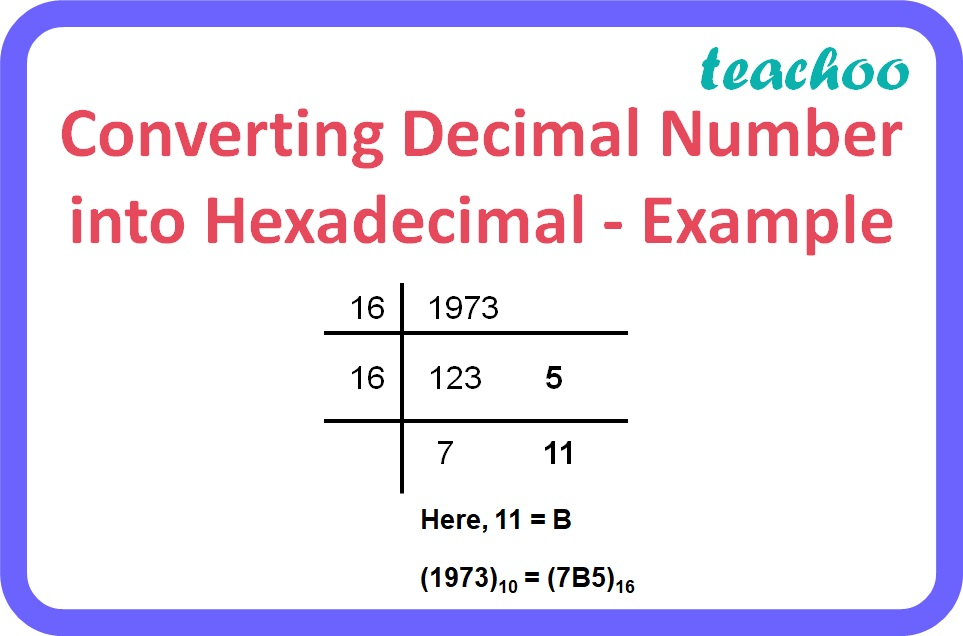Here, 11 = B

(1973) 10 = (7B5) 16

### 4. Binary to Decimal conversion

To obtain the decimal equivalent of a binary number, individual digits of binary number should be multiplied by powers of 2 starting with the rightmost digit multiplied by 2 0 , second last digit multiplied by 2 1 , third last digit multiplied by 2 2 and so on up to the leftmost digit. For digits after the decimal point, the leftmost digit should be multiplied by 2 -1 and the next digit by 2 -2 and so on up to the rightmost digit.

Example:

(101.01) 2

= 1 x 2 2 + 0 x 2 1 + 1 x 2 0 + 0 x 2 -1 + 1 x 2 -2

=    4      +    0     +     1     +    0       + 0.25

=    (5.25) 10

### 5. Octal to Decimal conversion

To obtain the decimal equivalent of an octal number, individual digits of octal number should be multiplied by powers of 8 starting with rightmost digit multiplied by 8 0 , second last digit multiplied by 8 1 , third last digit multiplied by 8 2 and so on up to the leftmost digit. For digits after the decimal point, the leftmost digit should be multiplied by 8 -1 and the next digit by 8 -2 and so on up to the rightmost digit.

Example:

(127.34) 8

= 1 x 8 2 + 2 x 8 1 + 7 x 8 0 + 3 x 8 -1 + 4 x 8 -2

=   64  +  16   +  7  + 0.375  + 0.0625

=   (87.4375) 10

### 6. Hexadecimal to Decimal conversion

To obtain the decimal equivalent of a hexadecimal number, individual digits of hexadecimal number should be multiplied by powers of 16 starting with rightmost digit multiplied by 16 0 , second last digit multiplied by 16 1 , third last digit multiplied by 16 2 and so on up to the leftmost digit. For digits after the decimal point, the leftmost digit should be multiplied by 16 -1 and the next digit by 16 -2 and so on up to the rightmost digit.

For alphabets A to F, the corresponding codes should be multiplied with the respective power of 16.

Here,

• A = 10
• B = 11
• C = 12
• D = 13
• E = 14
• F = 15

Example:

(1A.2) 16

= 1 x 16 1 + 10 x 16 0 + 2 x 16 -1

=    16      +     10       + 0.125

=  (26.125) 10

### 7. Octal to Binary conversion

To obtain binary equivalent of an octal number, individual digits of octal number should be converted to binary in groups of three digits as given in the table below.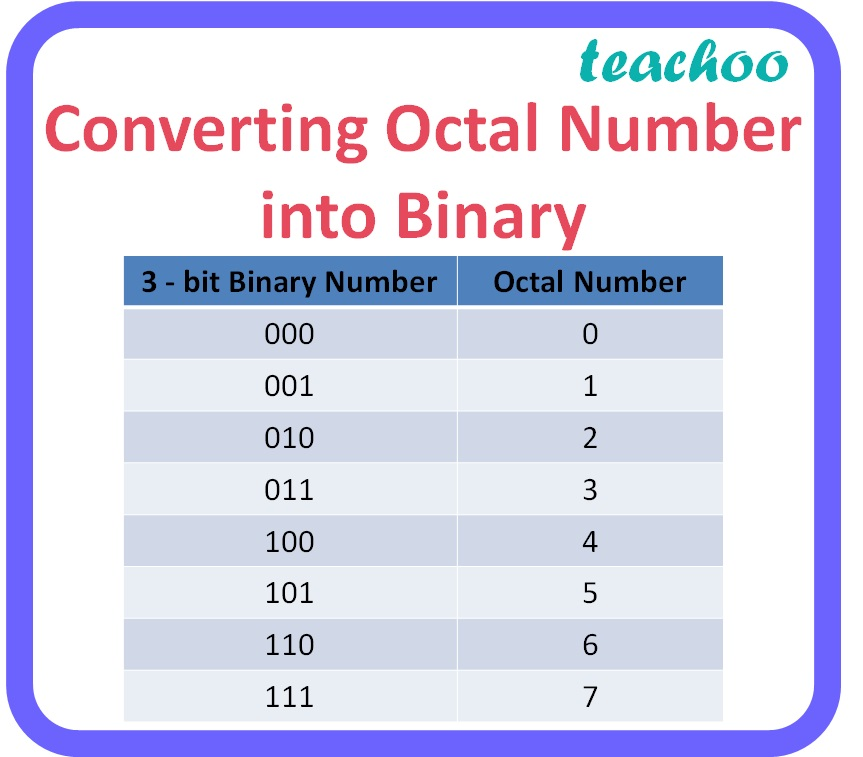Example:

(12.54) 8

1           2         .        5           4

001       010       .      101       100

= (1010.1011) 2

### 8. Hexadecimal to Binary conversion

To obtain the binary equivalent of a hexadecimal number, individual digits of the hexadecimal number should be converted to binary in groups of four digits as given in the table below.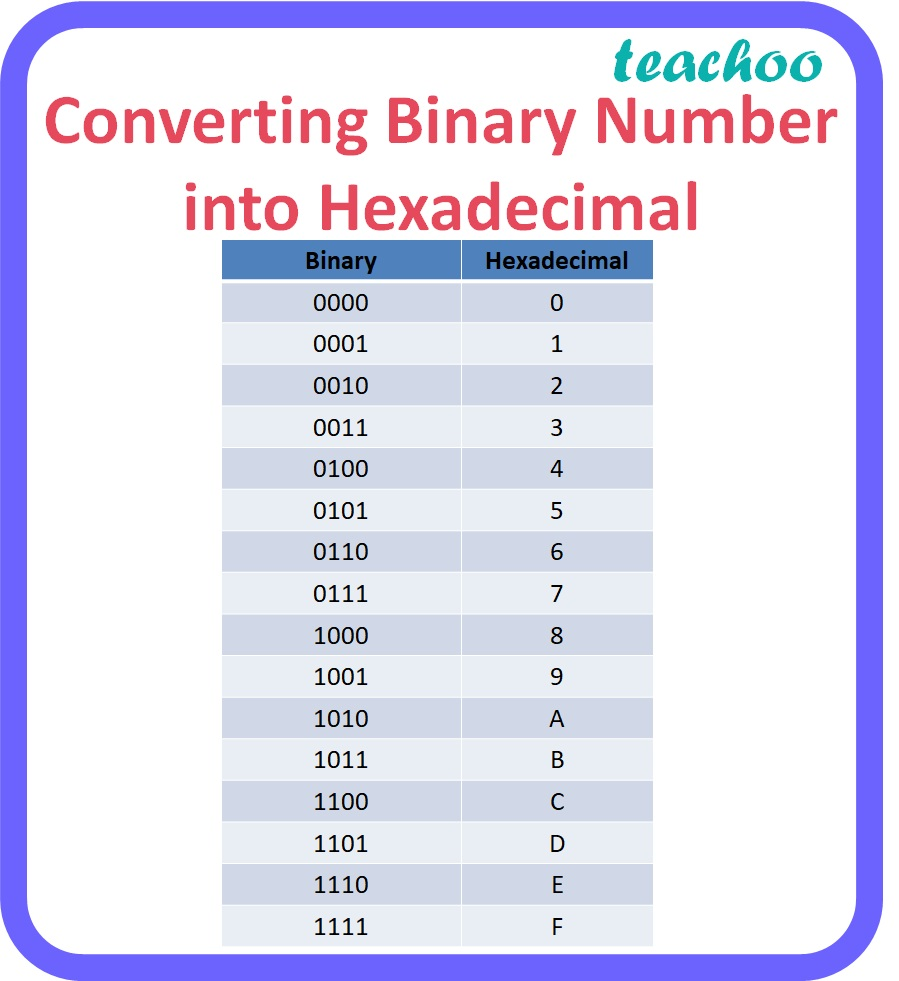Example:

(F2.A9) 16

F     2        .        A      9

1111 0010     .     1010 1001

= (11110010.10101001) 2

### 9. Binary to Octal conversion

To obtain the octal equivalent of a binary number, digits of binary number should be divided into groups of three digits starting from the rightmost digit for the integer part and starting from the leftmost digit for the fractional part. Convert each group of 3 digits to one octal digit as given in the table below.

Note: 1 or 2 zeroes can be added before the leftmost digit or after the rightmost digit to make a complete group of 3 bits.Example:

(1010011.11101) 2

 00 1 010 011 111 01 0

 1 2 3 7 2

= (123.72) 8

### 10. Binary to Hexadecimal conversion

To obtain the Hexadecimal equivalent of a binary number, digits of binary number should be divided into groups of four digits starting from the rightmost digit for the integer part and starting from the leftmost digit for the fractional part. Convert each group of 4 digits to one hexadecimal digit as given in the table below.

Note: 1,2 or 3 zeroes can be added before the leftmost digit or after the rightmost digit to make a complete group of 4 bits.Example:

(1010011.11101) 2

 0 101 0011 1110 1 000

 5 3 E 8

= (53.E8) 16

### 11. Octal to Hexadecimal conversion

To obtain the Hexadecimal equivalent of an octal number, first convert the octal number to its decimal equivalent and then convert the decimal number to its hexadecimal equivalent.

Example:

(115) 8

= 1 x 8 2 + 1 x 8 1 + 5 x 8 0

= 64 + 8 + 5

= (77) 10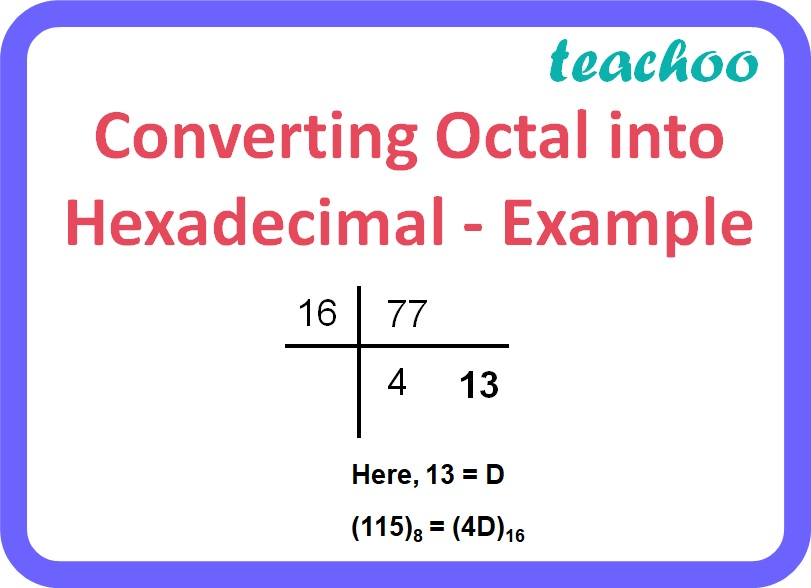### 12. Hexadecimal to Octal conversion

To obtain the Octal equivalent of a hexadecimal number, first convert the hexadecimal number to its decimal equivalent and then convert the decimal number to its octal equivalent.

Example:

(F29) 16

= 15 x 16 2 + 2 x 16 1 + 9 x 16 0

= 3840 + 32 + 9

= (3881) 10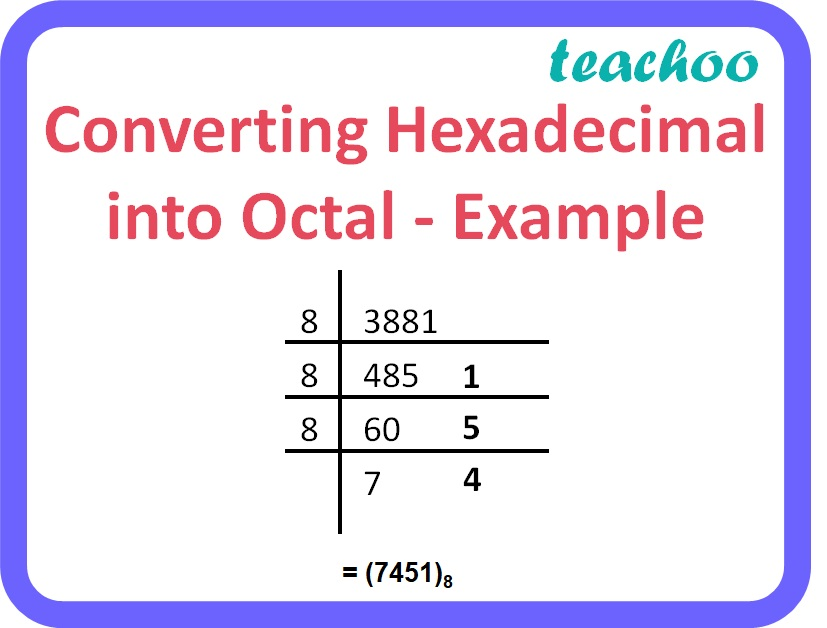= (7451) 8

Learn in your speed, with individual attention - Teachoo Maths 1-on-1 Class

### Transcript

Converting Decimal Number into Binary - Example Converting Decimal Number into Octal - Example (973)10 = (1715)8 Converting Decimal Number into Hexadecimal - Example (1973)10 = (7B5)16 Converting Octal Number into Binary Converting Hexadecimal into Binary Converting Binary Number into Octal Converting Binary Number into Hexadecimal Converting Octal into Hexadecimal - Example Converting Hexadecimal into Octal - Example